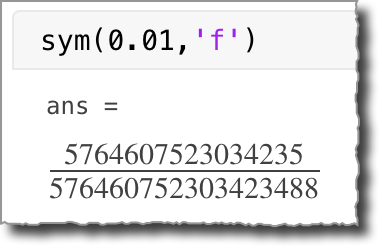# What is 0.01?

I was looking at these two numbers the other day:

$0.01$

$\frac{5764607523034235}{576460752303423488}$

It turns out they are very close, differing only by about 5 parts in a quadrillion.

A tech support case caught my attention on that day because MATLAB creator Cleve Moler (Numerical Computing with MATLAB, Experiments with MATLAB, Cleve's Laboratory, Cleve's Corner) responded to it, and I always enjoy Cleve's explanations.

The tech support case turned out to be related to understanding how floating-point numbers work. In part of his response, Cleve said, "1.e-2 is not one-hundredth; it is the floating point number nearest one-hundredth."

The floating-point number system used in most numerical computing applications, including MATLAB, is genius. It enables us to successfully pretend (most of the time) that we are working with the real numbers (ℝ). We type 0.01, meaning 1/100, without much thought.

Every now and then, however, it helps to be aware that the floating-point number system is really a finite-precision approximation of ℝ. Every number in this system is a base-2 fraction multiplied by a power of 2. Just as the rational number 1/3 does not have a finite-length representation in base 10, the rational number 1/100 does not have a finite-length representation in base 2. That means that when you enter 0.01 in a numerical application such as MATLAB or a spreadsheet program, the number stored by the computer is very, very close to 1/100, but not exactly equal to it.

You can get a sense of this by using format hex, which displays the underlying bytes of the floating-point number using hexadecimal notation:

format hex
a = 0.01

% Restore the normal format
format

a =

3f847ae147ae147b



You can see the repeating digit pattern ae147, with the final hexadecimal digit, b, representing ae147 rounded to b. To paraphrase Cleve's point, this number is not 1/100; it is a binary fraction, scaled by a power of 2, that is very close to 1/100.

I always find it interesting to use the Symbolic Math Toolbox to find out the exact value of a floating-point number. You can do this using the sym function with the 'f' option. According to the documentation, using this option returns a symbolic number that is a precise rational number equal to the floating-point value.

So, here is one representation of true value of the floating-point number you get when you type 0.01:

sym(0.01,'f')


ans =

5764607523034235/576460752303423488



This displays better using the Live Editor; here is a screenshot:I encourage you to take a look at Cleve's 07-Jul-2014 blog post on floating-point numbers. If your work involves any kind of numerical computing, it will be helpful to understand more about them.

Published with MATLAB® R2020a

|